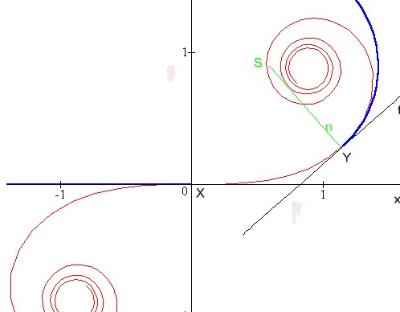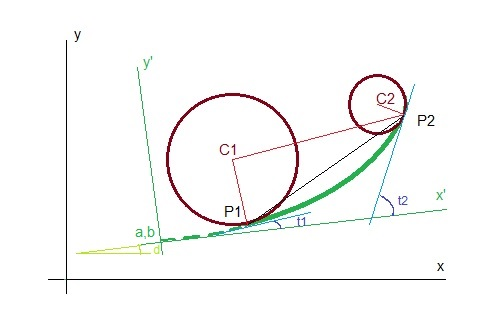# Euler spiral (Clothoid)

Clothoid is a curve whose curvature k changes linearly with its curve length (denote s or L). Clothoids are widely used as transition curves in railroad engineering for connecting and transiting the geometry between a tangent and a circular curve. Clothoid has the desirable property that the curvature k is linearly related to the arc length s. Although its defining formulas for coordinates are transcendental functions (Fresnel integrals), the important characteristics can be derived easily from equation k = s/A where A is constant. Some applications avoid working with the transcendental functions by proposing polynomial approximations to the clothoid, e.g. .Determine the length s of Euler spiral for transition between straight road and circular arc of radius r = 9 m. Solution: Curvature of a bend must be the same as curvature of a clothoid, i.e. and s = 16 m.

## Clothoid k = s/A

Determine an angle length α between the tangent of Euler spiral at s = 16 m and x-axis. Solution: Formula for direction part of a clothoid. and α = 50,92°.

## Clothoid between two circumferencesCircumferences must be tangent to clothoids, and radius of curvature must be the same at each tangent point.